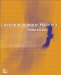# A.2 Numbers

## A.2 Numbers

Real numbers are numbers that can take a positive, negative, or zero value. For example:

• -400, -20, -11.75, -3, 0, +1, +3.76, +1000

Integers are whole numbers, positive or negative, that have no fractional parts. For example:

• -400, -20, -11, -3, 0, +1, +3, +1000

Rational numbers are fractional numbers and may be positive or negative. Rational numbers include fractions that are less than one, those that are greater than one (so-called improper fractions). Formally stated, rational numbers have the form a/b, where a and b are integers, b cannot be zero, and there are no common factors (i.e., 4/6 should be reduced to 2/3). Note that b can equal 1, so all integers, including zero, can be seen as rational numbers with a value of b equal to 1. For example:

• -7/2, -1/4, 0/1, +1/8, +1/4, +3/2, +16/1

Irrational numbers are a form of rational numbers where a and b are non-integer. Irrational numbers can be produced in a number of ways; for example, π is irrational, and the square root of any prime number is irrational (e.g., 2 or 13). For example:

• 2.2360679, 3.1415926

A Prime number is a whole number that is greater than 1 and has no common factors other than 1 and itself (i.e., it cannot be divided by two integers that are greater than 1). Every natural number greater than 1 is either prime or can be expressed as a product of primes (e.g., 28 = 2 × 2 × 7). Prime numbers have many useful applications in cryptography, as discussed in Chapter 15. Examples include:

• 2, 3, ..., 17, 19, 23, ..., 47, ..., 101, ..., 1093,

Infinity is itself not a number. In effect, it is an unbounded value; we may therefore use the phrase infinitely large or infinitely small to represent inconceivably large or small values respectively. The term infinitesimal means a value whose limit is zero. Infinity is normally represented by the symbol .ActionScripting in Flash MX
ISBN: N/A
EAN: 2147483647
Year: 2001
Pages: 117